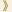HomeShaw Laureates2016Mathematical SciencesEssay

From antiquity the subject of geometry has been at the centre of mathematics. The ancient Greeks were fascinated by the subject and studied it extensively, giving us Euclidean geometry. The modern view of geometry dates to the middle of the 19th century, when Gauss introduced and developed the theory of curved surfaces. He was followed by Riemann who constructed the theory of higher-dimensional curved spaces, now called Riemannian geometry. Their work began a period of flowering of geometry, and our present-day understanding of the subject emanates directly from their work.

Nigel Hitchin is one of the most influential geometers of our time. The impact of his work on geometry and on many of the allied areas of mathematics and physics is deep and lasting. On numerous occasions, he has discovered elegant and natural facets of geometry that have proven to be of central importance. His ideas have turned out to be crucial in areas of mathematics and physics, far removed from the context in which he first developed them. By exploring ignored corners of geometry, Hitchin has repeatedly uncovered jewels, many more than described below, that have changed the course of developments in geometry and related areas, and changed the way mathematicians think about these subjects.

In the modern period geometry has flourished and expanded greatly. With the development of topology (which may be described as the study of shapes, allowing deformation but not tearing) as an independent discipline in mathematics around 1900, the purview of geometry expanded to include auxiliary objects associated with spaces. A prime example is gauge theory, which is the study of the geometry of certain curved structures over spaces known as fiber bundles.

Geometry has close connections to other parts of mathematics such as representation theory, which is related to the study of symmetry, differential equations and dynamics, and number theory. It has had a profound impact on topology. Its connections to physics are long-standing as well: Einstein formulated general relativity in terms of the geometry of curved four-dimensional spacetime, essentially in the form that Riemann had introduced. More recently, the standard model of particle physics is formulated using the geometry of gauge theories, and much of theoretical high-energy physics beyond the standard model is formulated geometrically.

While Hitchin has introduced many important concepts and techniques in geometry, one of his most influential works is his study of Higgs bundles over a Riemann surface. The parameter space of all Higgs bundles over a fixed surface is itself a fiber bundle over that surface. On these fiber bundles Hitchin defined a natural function that produces an algebraically completely integrable Hamiltonian system on the space. The result is what is now called the Hitchin fibration of the space of Higgs bundles. One aspect of these spaces is that they give fundamental examples of important objects known as hyperkahler manifolds. But the impact of Hitchin fibrations is not limited to Higgs bundles and hyperkahler manifolds. Hitchin fibrations and his quantization of them are cornerstones of the construction of a modern branch of representation theory called ‘the geometric Langlands program’. In addition, they are a foundational ingredient in Ngo’s recent Fields-Medal-winning work in the theory of automorphic forms and number theory. In a related work, Hitchin used this theory to construct projectively flat connections over the moduli spaces of Riemann surfaces, which had been predicted by Witten's analysis of Chern-Simons theory, a three-dimensional topological quantum field theory. Hitchin fibrations have also served as a point of departure for physicists in their studies of certain four- and six-dimensional quantum field theories. The story of Hitchin fibrations is a perfect example of Hitchin’s ability to find appealing and natural questions that had been previously overlooked but whose answers are of fundamental importance across a wide spectrum of mathematics and physics.

Hitchin's work with Atiyah, Drinfeld and Manin brought mathematics to bear on a question of fundamental importance in physics, namely the description of the moduli space of instantons on four-dimensional Euclidean space. Their approach using linear algebra, even now, thirty years later, forms a basis for much work on the study of instantons in both mathematics and theoretical physics, and serves as an important bridge between mathematics and physics.

The Kobayashi–Hitchin conjecture relating algebro-geometric stability and solutions to the partial differential equations describing instantons on complex algebraic surfaces was one of the first examples of a close relationship between the notion of stability in algebraic geometry and solutions of non-linear partial differential equations. This fundamental principle has reoccurred in various forms in many areas of mathematics.
The influence of Hitchin's work across the wide sweep of geometry and its applications to allied fields will be felt far into the future. His many achievements richly merit the award of the Shaw Prize in Mathematical Sciences.

27 September 2016   Hong Kong